Himanshi Singh — Updated On March 12th, 2021

## Introduction

Ensembling is nothing but the technique to combine several individual predictive models to come up with the final predictive model. And in this article, we’re going to look at some of the ensembling techniques for both Classification and Regression problems such as Maximum voting, Averaging, Weighted Averaging, and Rank Averaging.

Note: If you are more interested in learning concepts in an Audio-Visual format, We have this entire article explained in the video below. If not, you may continue reading.

## Overview of Ensemble Technique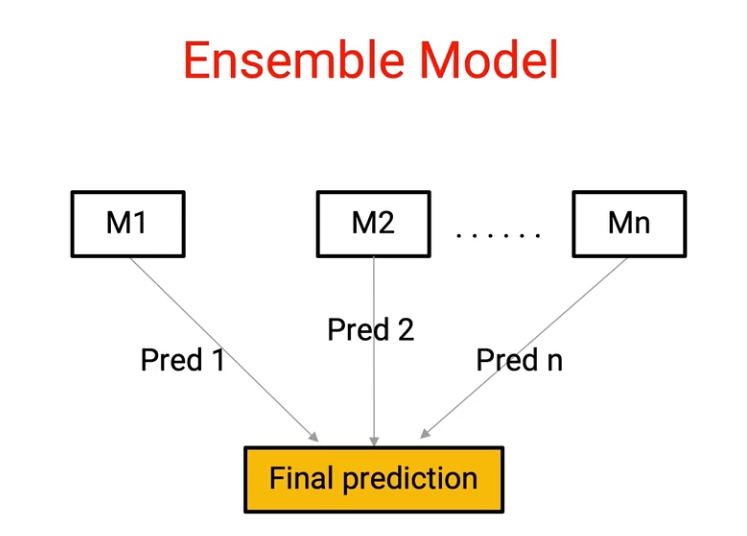Here we have M1 to Mn individual models which are giving predictions from Pred 1 to Pred n respectively. And we combine them to come up with a single model. Now, the question is, how do we do this combination and there are several techniques to do this, and this is where the art of ensembling comes into the picture. So let’s start by understanding some of these basic techniques.

And in order to understand them, we’ll be using the Titanic survival example, where we have details about individual passengers who were traveling on Titanic and we have to predict which customer would survive the Titanic tragedy.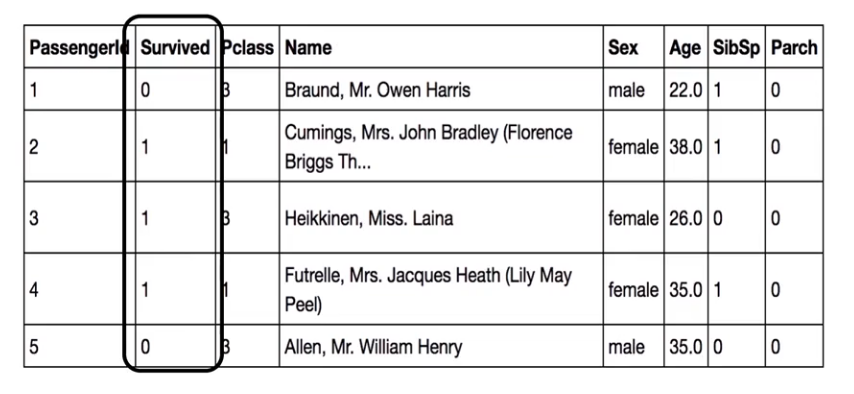So let’s say we have three models, M1, M2, M3, which are based on different techniques such as first could be based on logistic regression and second could be based on K nearest neighbors and so on.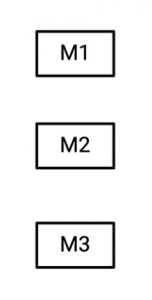So let’s say there are these three models which are giving us three different outcomes. So this table shows your different outcomes from each of these models.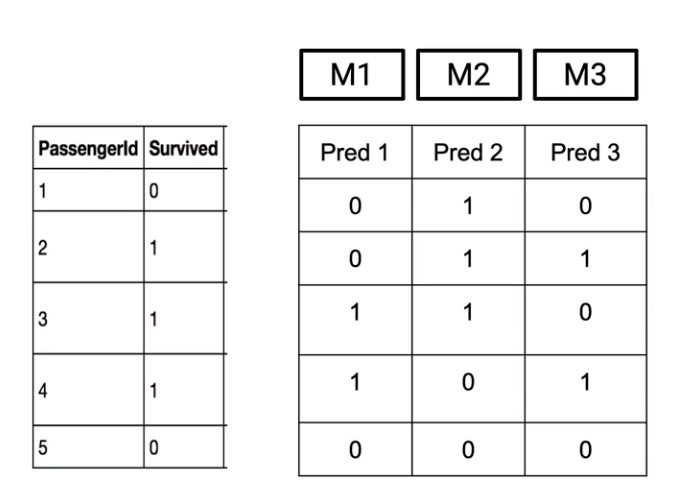So what this means is the first Model(M1) says that the passenger with Passenger ID 1 did not survive the Titanic tragedy(indicated by 0). Whereas the second model(M2) says he or she survived(indicated by 1). And third model(M3) again says that the passenger did not survive(indicated by 0). So let’s say these three models have their individual predictions and we want to combine them to come up with a final prediction.

So how do you do that? Well, the simplest technique is to just take final outcomes from each of these models and take the maximum voting of each of them.

## Ensemble Technique: Max Voting

So what do I mean by maximum voting? Well, whatever my models tell me the most is what I’ll go ahead with. So considering the car analogy, if I want to choose between let’s say Model A and Model B, if multiple people are recommending me Model A over Model B, I’ll go with Model A.

So the same rule applies here. If more models are telling me that a pass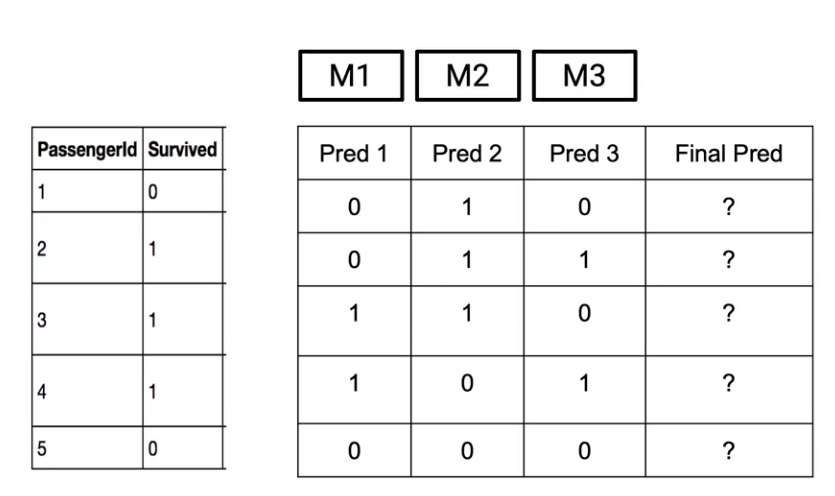enger would survive, I will consider the passenger survived. Whereas if more models are telling me that the passenger did not survive, I will predict that the passenger did not survive-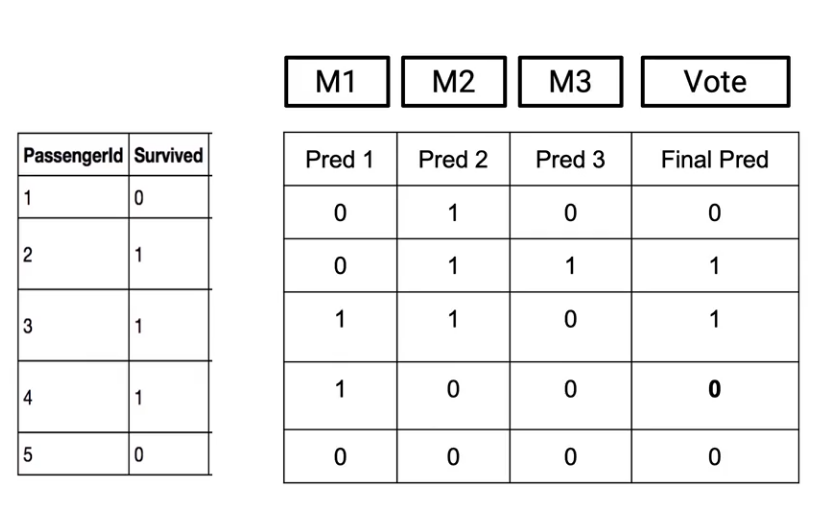So here in this case, so for example, passenger one-two of my models said that the passenger would not survive. So my final prediction is 0. On the other hand, in the case of Passenger ID 2, since M2 and M3 were saying that the passenger would survive, I’ll make my final prediction as 1.

So, Max Voting is the way in which I think the outcome from individual models and just take a vote.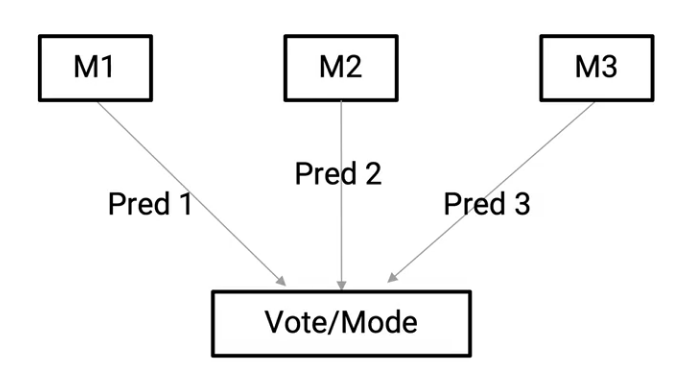Now, this cannot apply to the regression problem where we have to actually predict the continuous variable. So what do you do in this case? Well, in order to do this, let’s actually go through the example.

## Ensemble Technique: Averaging

So, again, let’s have three different models M1, M2, and M3 which are giving us individual predictions.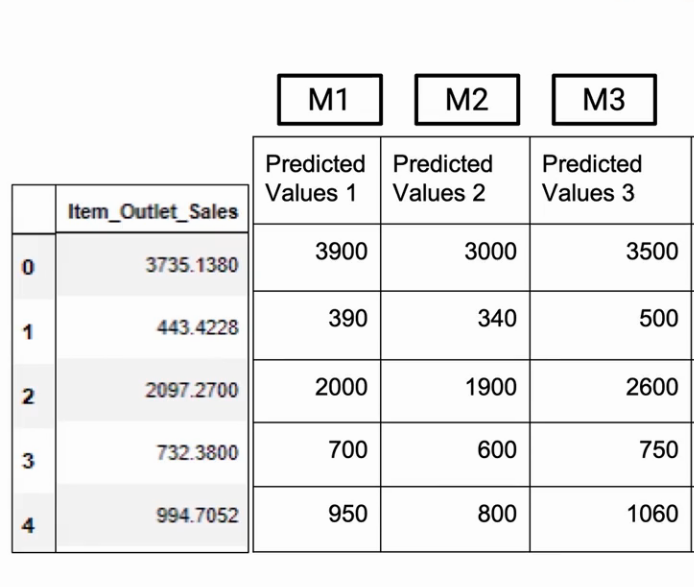And what I need to do is make the final prediction. And the nearest analogy I can have with Max Voting is average. So I’ll just take an average of whatever my individual models are telling.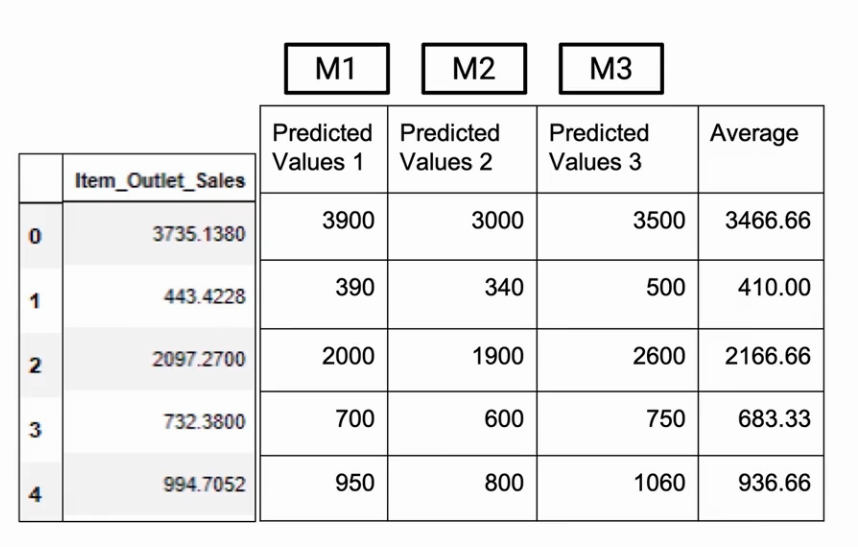So in this particular example for Row 0, we got an average of 3466.66 . So that would be my prediction.

## Ensemble Technique: Weighted Averaging

Now, if you think about it, what I’m doing is I’m not taking into consideration the accuracy of individual models. I’m giving each of these models equal weightage, which is not a very prudent strategy because if I know that a model is performing better, I would want to give it higher weightage.

Going back to the Car example, if I know that a person has more knowledge about cars, I would like to give his or her recommendation a higher rate.

So coming back, let’s say that these three models M1, M2, and M3 have an R-square on validation of 0.6, 0.4, and 0.7 respectively.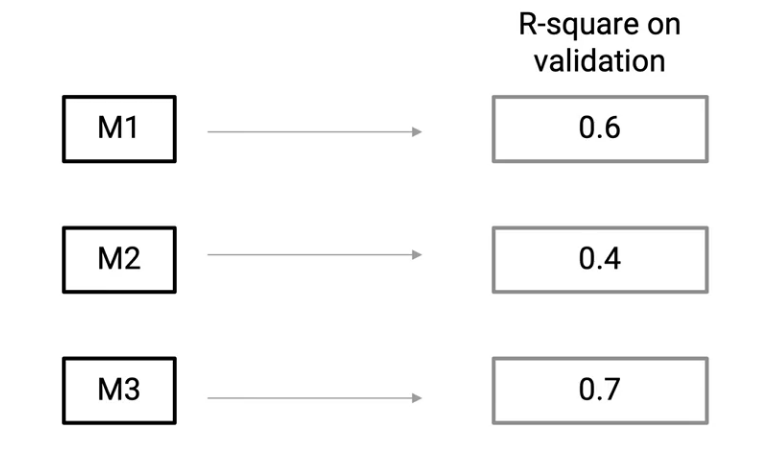So what does this mean? This means that M2 is not performing great as compared to M1 and M3. So M3 is my best model. And M2 is my worst model.

Now, if I have to somehow combine them more intelligently, what I can do is I can give them some sort of weights and these weights would be representative of how much weight do I give to their predictions, since in this particular case, my model M2 is not the best model, I’ll give it a lower weight whereas M1 and m3 are better models. I’ll give them higher weights. So let’s give them the following weights-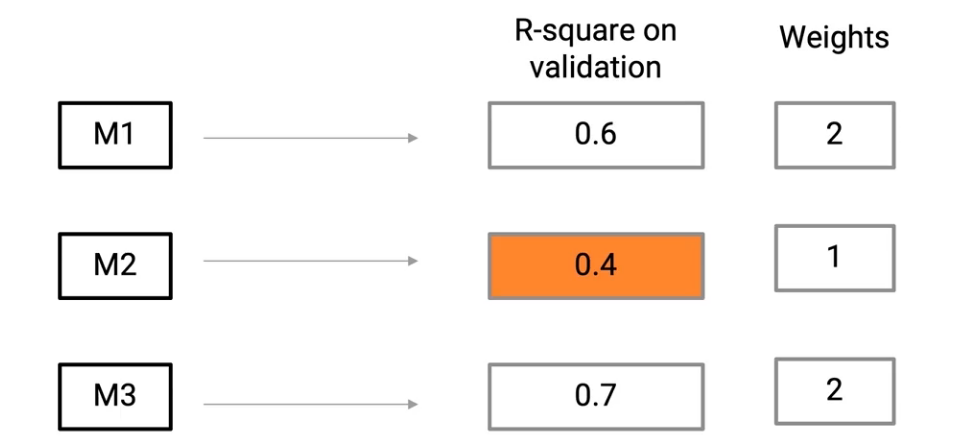What I would do now is I’ll basically multiply these weights to individual predictions and then take their mean. So M1 predictions which are, 3900, 390, etc, we’ll multiply them by a factor of 2-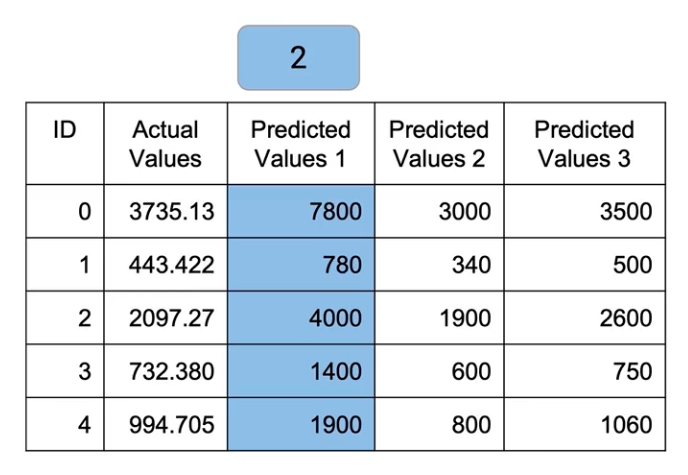After Multiplying the values of M1 by 2

Similarly, We’ll multiply M2 by a factor of 1. So basically no values change there. And M3, we’ll multiply by a factor of 2 again,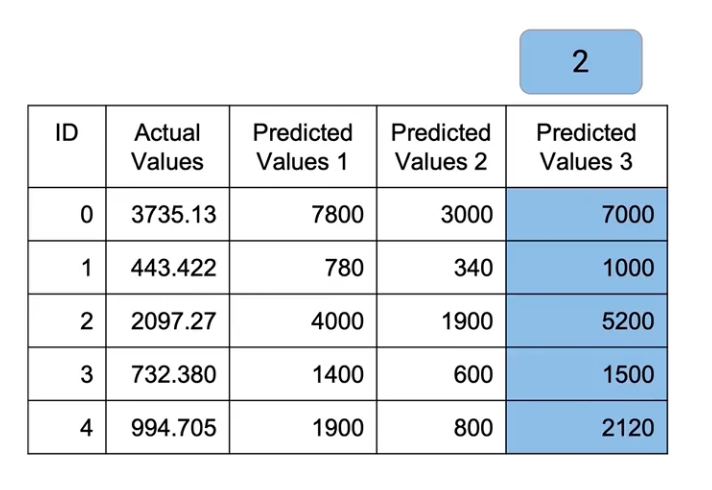and now I take an average of all of these by dividing it by 5(2+1+2)-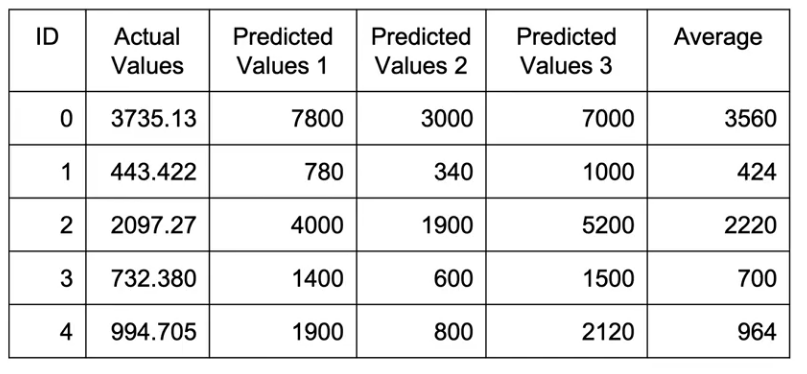So what I’ve done is I’ve taken weighted average from these models, but the weights were decided by me. That should give me better results because I understand that these models work better and I’ve given them more weight.

## Ensemble Technique: Rank Averaging

Now is there any other way to do it instead of me deciding how much weight to give to each mode? And that is where Rank Averaging comes into play. So what we’ll do is we give ranks to individual models-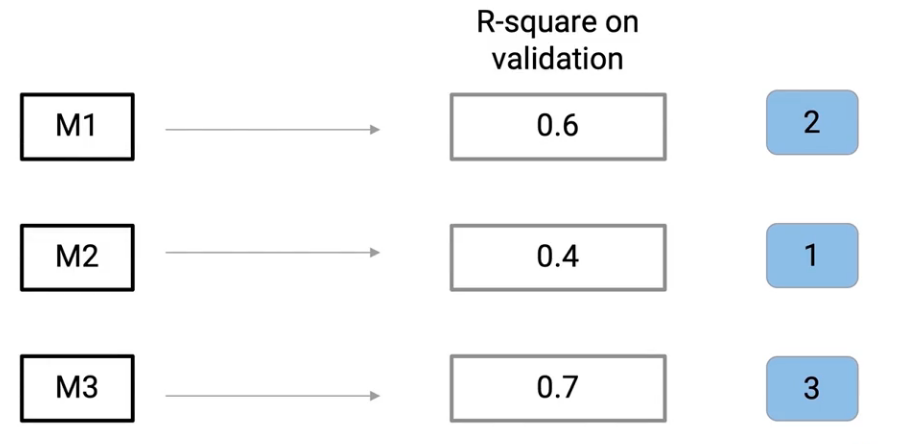So a quick thing to remember over here is that I’m giving rank 1 to my worst-performing model. So rank 1 is to the model which performs the least, rank 2 is the next best model, and rank 3 is the next best model. So I give these ranks to each of these models and then I take weights from these ranks. So I basically sum up these ranks and divide each of these ranks by that total value.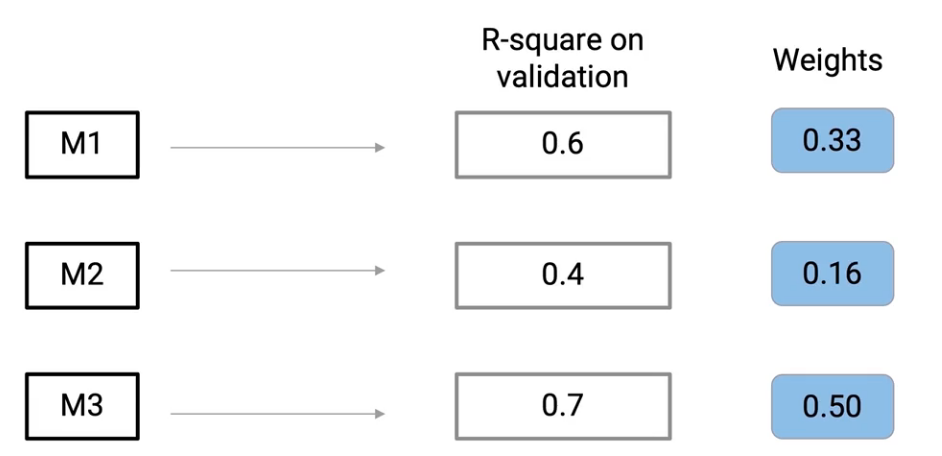For example- In the case of Model 2, we’ll divide 1 by the sum of 1+2+3 = 6. So the weight for Model 2 comes down to 1/6 = 0.16. Similarly, I come up with weights for each of these models and then I multiply those weights by individual models.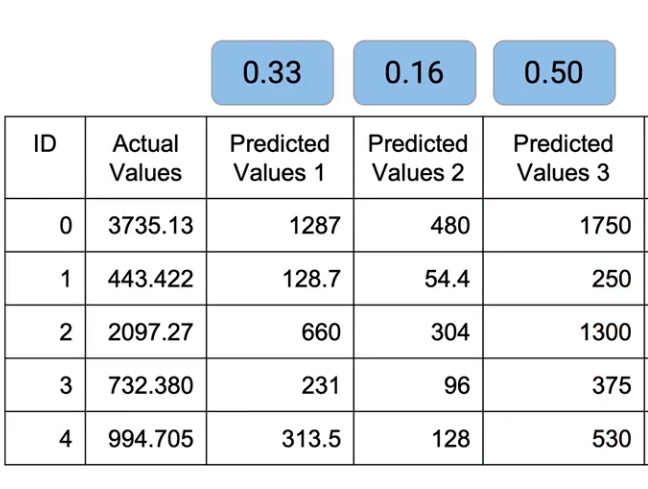So, all the predicted values of Model 1 get multiplied by 0.33. Similarly,  M2 and M3 are multiplied by 0.16 and 0.5 respectively. And then we have to sum up all of these values-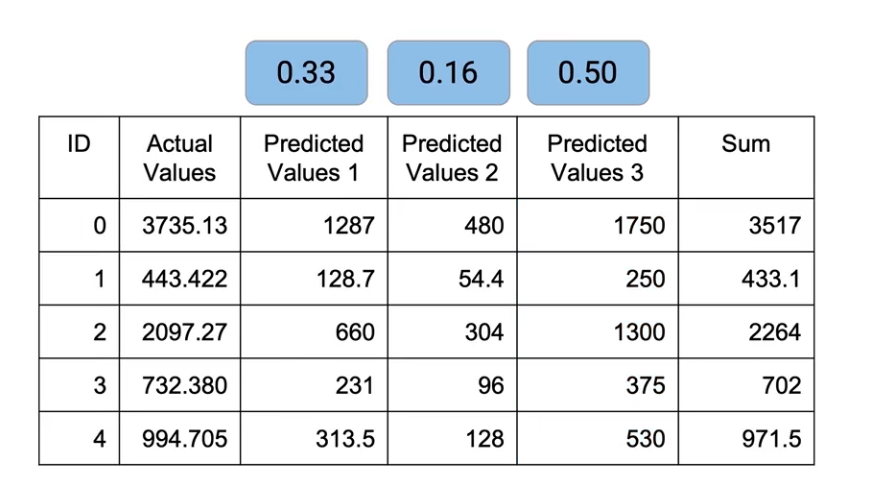Now, remember, I don’t need to divide because this is not mean. I’ve already done the normalization at the time of taking weights, so I just need to sum them up. And this is what I get as the final outcome. And this is Rank Averaging.

## End Notes

So there are various techniques that we saw which can be used to combine the results of these models, starting from Max Voting. Then we looked at Averaging, Weighted Averaging, and Rank Averaging. And using these techniques, we can cover classification models as well as regression models.